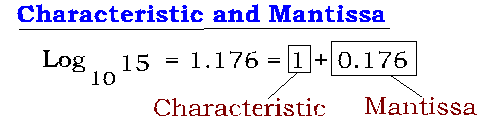## Characteristic and Mantissa in Logarithms

Algebra > Logarithms > Characteristic and Mantissa

8. Characteristic and Mantissa:

Consider a number N > 0.

Then, let the value of log 10 N consist of two parts:

One an integral part, the other – a proper fraction.

The integral part is called the Characteristic and the fractional or the decimal part is called the Mantissa.

For example,

log 10 15 = 1.176 = 1 + 0.176In 1.176, the Integral part is “1” and the decimal or fractional part is “0.176”

Therefore, for the number log 10 15:

the Characteristic is 1and the Mantissa is 0.176

Now, let us discuss Characteristic of logarithms of numbers greater than 1 and less than 1

9. How to find the Characteristic of the logarithm of a Number:

1. Characteristic of the logarithm of a number greater than 10:

Consider the following logarithms of different numbers (all expressed to base 10)

log 15 = 1.176 = 1 + 0.176

log 250 = 2.397= 2+0.397

log 4000 = 3.602=3+0.602

log 50000 = 4.698=4+0.698

look at the four examples above closely. Observe closely the relation between the number of digits in the number on the left (whose logarithm is being written) and the digit before the decimal point in the number in the center.

What do you see?

The number of digits in the number on the left (15, 250, 4000 and 50000) is one more than the digit before the decimal point in the decimal number in the center (i.e., the logarithm of the number on the left)

2. Characteristic of the logarithm of a positive number less than 1

In the following examples, we consider logarithms of numbers less than 1(all numbers are expressed to base 10)

log 0.5 = -0.301 = -1 + 0.699

log 0. 08 = -1.096 = -2 + 0.904

log 0.005 = -2.3010 = -3 + 0.699

From the discussion in Characteristic and Mantissa, we must write an integer for the characteristic. And the mantissa cannot be a negative number

Therefore, in each of the examples above, the logarithmic values (on the right side) of the numbers (on the left) are converted into a sum of :

a negative integer and a positive proper fraction.

Therefore,

log 0.5 = -0.301 = -1 + x      {where x is a proper fraction to be found}

which gives x = 0.699

(do not express -0.301 = -2 + x , for, in that case x = 1.699, which is not a proper fraction)

Therefore, log 0.5 = -1 + 0.699

Similarly,

log 0. 08 = -1.096 = -2 + x

x = 2 – 1.096 = 0.904

therefore, log 0.08 = -2 + 0.904

Note: 1. The characteristic of the logarithm of a number must be an integer, negative or positive

2. the Mantissa of the logarithm of a number must be a positive proper fraction.

From the above examples, we have:

log 0.5 = -1 + 0.699

log 0.08 = -2 + 0.904

log 0.005 = -3 + 0.699

from the two examples above, what is the relation between the number of zeroes to the right of the decimal in the number on the left and the negative integer on the right.

It is like this:

In log 0.5, the characteristic is -1; the number of zeroes to the right of the decimal is 0,

In log 0.08, the characteristic is -2; the number of zeroes to the right of the decimal is 1,

In log 0.005, the characteristic is -3; the number of zeroes to the right of the decimal is 2.

So, the relation is now clear.

The characteristic (in the logarithm of a number) is one more than the number of zeroes to the right of the decimal in a positive number less than 1